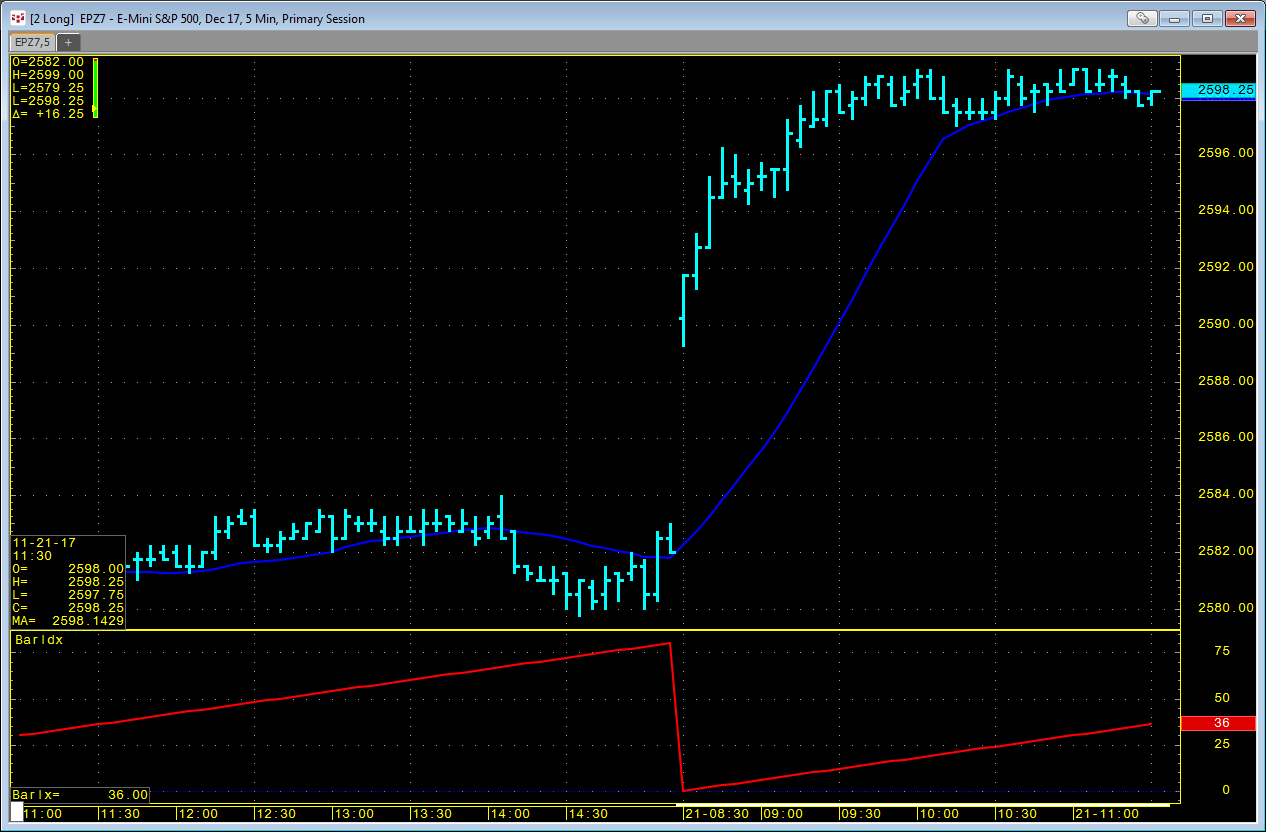# Pulling Market Data by Time Using Bar Index Function

You can use LocalHour and LocalMinute functions in the RTD formula to pull in the open price for a market using a 5-minute bar at 12:00. This technique was detailed in a previous article here.If you are pulling in a large amount of data using the above functions, then it can create a heavy load on CQG and Excel. A better way is to use the Bar Index function. There is a study you can add to a chart called Bar Index (BarIdx). This study simply counts the number of bars based on one of the following choices:

• Start of Day = number of bars since the start of trading day
• End of Day = number of bars left in trading day
• Start of Session = number of bars since start of session
• End of Session = number of bars left in session

The output is BarIx. We can use this in an RTD formula:

`=RTD("cqg.rtd",,"StudyData", "Open(EP) when Barix(EP,reference:=StartOfSession)=42", "Bar", "", "Open","5","0","PrimaryOnly",,,"False","T","EveryTick")`

If I add the BarIdx study to a 5-minute chart of the E-mini S&P futures contract (Symbol EP) set to the Primary Session, I will see that the index value of 42 is the 12:00 bar. Therefore, the above RTD formula gives me the open of the 12:00 5-minute bar for the E-mini S&P futures contract. If the current time is before 12:00, then the RTD formula pulls the previous session's 12:00 open. We can force it to be blank by including a date. If today is the 21st, then the cell is blank until 12:00 today.

`=RTD("cqg.rtd",,"StudyData", "Open(EP) when LocalDay(EP)=21 and Barix(EP,reference:=StartOfSession)=42", "Bar", "", "Open","5","0","All",,,"False","T","EveryTick")`

The above formulas also enable you to pull in the open at 12:00 for a day earlier in the month, such as the 6th by replacing 21 with 6.

You can also pull in a study value using the BarIx function. For example, here is the RTD formula to pull in a 21-period simple moving average using the primary session on the 20th.

`=RTD("cqg.rtd",,"StudyData","MA(EP,MAType:=Sim,Period:=21,InputChoice:=Close) When LocalDay(EP)=20 and Barix(EP,reference:=StartOfSession)=42","Bar","","Close","5","0","PrimaryOnly",,,"False","T","EveryTick")`

A simple sample Excel file is provided.